•5星
198KB weixin_48549810 2021-01-06 14:45:40
•5星
1KB weixin_42696271 2021-09-11 08:47:59
•5星
251KB weixin_42696333 2021-09-10 21:01:43
•4星
2KB weixin_42696333 2021-09-10 21:15:20
•5星
16KB weixin_42696333 2021-09-10 17:35:10
•5星
159KB weixin_54707168 2021-06-18 15:40:57
•5星
14KB weixin_42696271 2021-09-11 03:41:54
•5星
10KB ailemony 2020-12-13 15:34:25
•5星
7.84MB yunlong3267 2021-07-23 16:49:56
•5星
19KB qq_32858211 2021-08-09 17:12:28
• 【机器学习】参数估计,非参数估计,监督学习,无监督学习 机器学习 python 人工智能 深度学习 java

参数估计 已经知道观测数据符合某些模型的概率下，我们可以利用参数估计的方法来确定这些参数值，然后得出概率密度模型。这个过程中用到了一个条件，就是概率分布符合某些模型这个事实。在这个事实上进行加工。 ...

参数估计

已经知道观测数据符合某些模型的概率下，我们可以利用参数估计的方法来确定这些参数值，然后得出概率密度模型。这个过程中用到了一个条件，就是概率分布符合某些模型这个事实。在这个事实上进行加工。

个人理解：概率密度函数形式已知，求出形式中的参数。

非参数估计(无参密度估计)

实际中，概率密度形式往往未知，往往有多个局部最大；对于高纬度样本，一些高纬度的概率密度函数可以用低纬度密函数的乘积表示的假设通常也不成立。所以概率密度函数形式未知，只能用别的方法求概率密度。

概率密度估计--参数估计与非参数估计

我们观测世界，得到了一些数据，我们要从这些数据里面去找出规律来认识世界，一般来说，在概率上我们有一个一般性的操作步骤

1. 观测样本的存在

2. 每个样本之间是独立的

3. 所有样本符合一个概率模型

我们最终想要得到的是一个概率密度的模型，有了概率密度模型以后，我们就可以统计预测等非常有用的地方，因此，首要任务是找出一些概率分布的概率密度模型。

我们来分析一下上面的三个步骤，第一第二都很好解决，关于第三点，我们可以有不同的处理方式

如果我们已经对观测的对象有了一些认识，对观测的现象属于那种类型的概率密度分布已经了解了，只是需要确定其中的参数而已，这种情况就是属于参数估计问题。

如果我们研究观测的对象，也很难说这些观测的数据符合什么模型，参数估计的方法就失效了，我们只有用非参数估计的办法去估计真实数据符合的概率密度模型了。

因此，本文主要讨论 参数估计和非参数估计问题

1. 参数估计

对我们已经知道观测数据符合某些模型的情况下，我们可以利用参数估计的方法来确定这些参数值，然后得出概率密度模型。这个过程中用到了一个条件，就是概率分布符合某些模型这个事实。在这个事实上进行加工。

一般来说，参数估计中，最大似然方法是最重要和最常用的，我们重点介绍参数估计方法

我们在《无基础理解贝叶斯》中已经讲过似然性，那么我们就可以先写出似然函数。

假设有N个观测数据，并且概率模型是一个一维的高斯模型，用f(x)表示高斯模型，参数待定，因此我们可以写出似然函数

L(x1,x2,...xn) = f(x1,x2,...xn) = f(x1)*f(x2)*......*f(xn),第二个等式用到了样本之间是独立性这个假设(上面提到的一般步骤的第二条)

然后把对似然函数取对数

logL(x1,x2,...xn) = log(f(x1)*f(x2)*......*f(xn)) = log(f(x1)) + log(f(x2))+......+log(f(xn))

我们既然提到了极大释然方法，那就是要求出使得logL(x1,x2,...xn) 取最大值得参数。

因此对 logL(x1,x2,...xn) 求导等于0的参数就是符合要求的参数。

注意，如果似然函数求导有困难，通常我们会用迭代方法去求得这些参数，后面我们讲EM算法就是属于此类型

2. 贝叶斯方法

在我们谈到参数估计方法中，我们假定了参数是固定值，但是贝叶斯观点会人文，模型的参数值不是固定的，也是属于某种分布的状态。

因此我们做参数估计的时候其实是不准确的，因此贝叶斯方法会把参数的也作为一个概率考虑进来，然后再去观测。

我个人理解，这种方式也只能算是参数估计里面的一个变种而已

后验概率 ∝ 似然性 * 先验概率

先验概率，我们可以看成是待估计模型的参数的概率分布，后验模型是在我们观测到新的数据以后，结合先验概率再得出的修正的参数的分布

注意，如果似然函数的形式和先验概率的乘积有同样的分布形式的话，得到的后验分布也会有同样的分布模型

因此，人为的规定，如果先验概率与似然函数的乘积在归一化以后，与先验分布的形式上是一致的话，似然函数与先验概率就是共轭的，注意共轭不是指先验与后验的共轭

至于满足这个条件的共轭分布有很多种，二项分布与贝塔分布，多项式分布于狄利克雷分布等

后面有时间再更新一些贝叶斯方法相关的内容

3. 非参数估计

看过了参数估计后，我们知道，如果有模型的知识可以利用的话，问题就会变得很简单，但是如果没有关于模型的知识，我们怎么办？

回过头来看我们的目标，求出观测数据的概率密度模型。因此我们就会从概率密度这个定义开始分析，看有没有可以入手的地方。

概率密度，直观的理解就是在某一个区间内，事件发生的次数的多少的问题，比如N(0，1)高斯分布，就是取值在0的很小的区间的概率很高，至少比其他等宽的小区间要高。

我们把所有可能取值的范围分成间隔相等的区间，然后看每个区间内有多少个数据？这样我们就定义出了直方图，因此直方图就是概率密度估计的最原始的模型。

直方图我们用的是矩形来表示纵轴，当样本在某个小区间被观测到，纵轴就加上一个小矩形。

这样用矩形代表的模型非常粗糙，因此可以用其他的形状来表示，进一步就是核密度估计方法，这个后面会有一个翻译文章来具体讲解

基本上，参数估计和非参数估计是概率模型里面用的非常多的基本概念，希望自己在后面忘记的时候还能想起来曾经写过的东西

展开全文weixin_42319496 2021-08-04 07:53:50
• 非参数估计 机器学习

出发点：我们以前在参数估计中都是先假设样本点的分布有一个概率密度函数形式，比如高斯分布，然后从样本中估计参数。但是，有可能根本不是，所以也有非参数法研究的必要，比如我们中学就学过频率分布直方图，但是...

引入

出发点：我们以前在参数估计中都是先假设样本点的分布有一个概率密度函数形式，比如高斯分布，然后从样本中估计参数。但是，有可能样本点的分布根本不是高斯分布，那么我们的结果就错了。

本文提出的非参数法讲究不需要先假设样本服从一个什么分布，而是直接从样本中统计得到，比如频率分布直方图。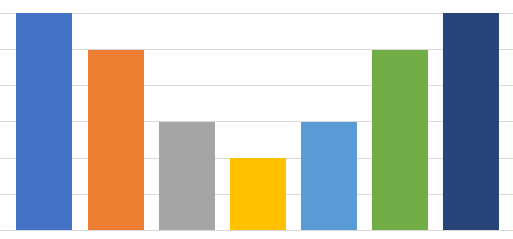这种情况下：我们要记录的是每一组的边界和每一组的数量，然后就能描述我们的样本分布。

理论上，如果我们样本足够多，同时将频率分布直方图组距设置得特别小，组特别多，这就是在逼近样本点的真实概率密度函数。

一般地，样本足够多的时候其可以表示出任意类型的分布！

基础知识

既然非参数法这么好，下面开始介绍基础建筑。这个很好理解，R是指这个一定区域， p ( x ′ ) p(x^{'}) 是概率密度函数。就有点像二维的情况。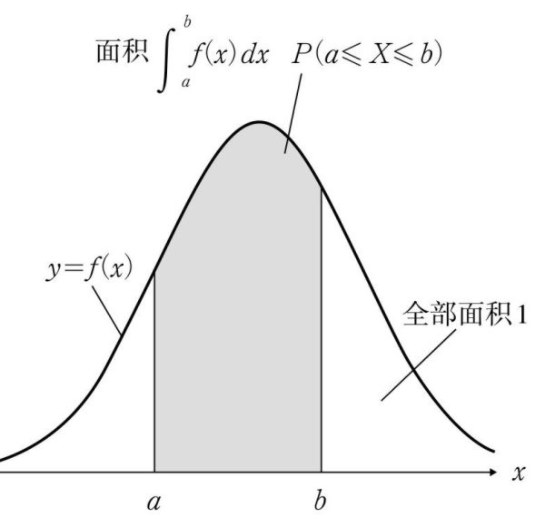所以上上幅图求的是样本落在R空间的概率。
我们继续下一个知识点。该区域等概率密度，那么不就可以算该区域的概率密度了嘛!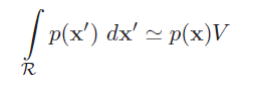而这个由最前面的概念知道，这个是在样本落在空间R中的概率，我们假设R里面有k个样本，整个空间有n个样本，那么样本落在空间R中的概率就是 k n \frac{k}{n}
从而求得空间R中的概率密度函数为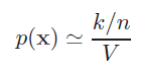我们要估计样本的概率密度函数，但是这个V如何选择呢？这就有点像你要画个频率分布直方图，组距选择多大呢?显然，我们都认为：如果样本数无穷多个，那么我们空间V就选小点，这样就可以表示得更加精确。就像下面这样：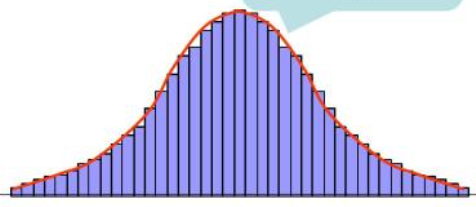因此：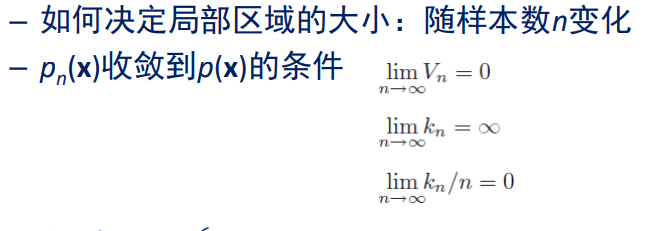解释：上面的 p ( x ) p(x) 表示的是真实概率密度函数，我们希望可以逼近它。
上面都是理论，下面探讨细节。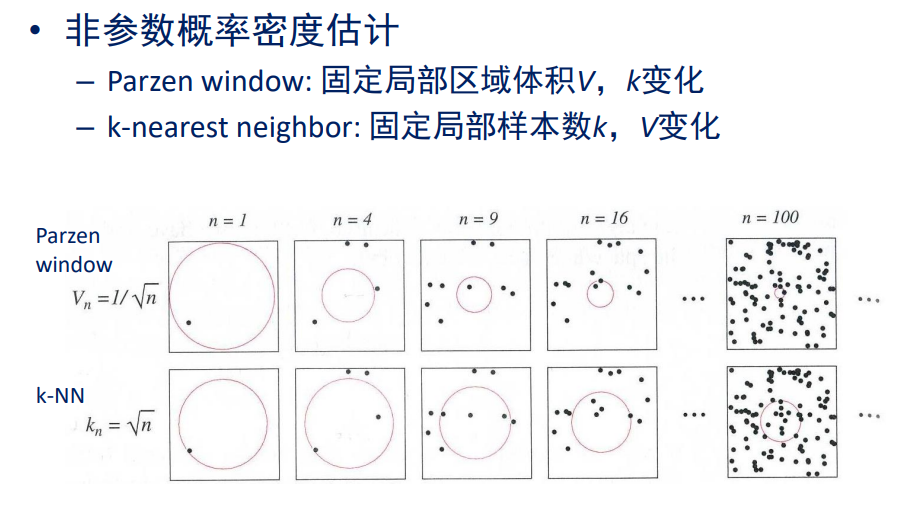上面的大家好好看一下就行，没懂也没关系，后面会展开介绍。

Parzen窗估计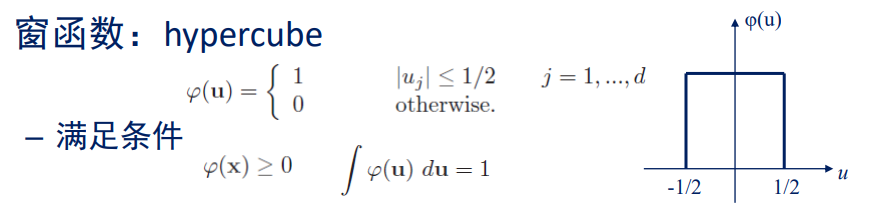解释：这里拓展到了高维的情况，但是低维也适用。样本空间是d维。
然后定义了一个窗函数，即在落在窗口内则函数值为1，否则为0。这个窗口在一维是一个关于原点对称的线段，长度为1；在二维是一个关于原点中心对称的正方形，面积为1；在三维是一个关于原点中心对称的正方体，体积为1。注：上面 h n h_n 是边长。
我们现在用上窗函数了，即对于任意一个样本点 x i x_i ，只要满足向量 x − x i x-x_i 的某一个维度 < = h n / 2 <=h_n/2 ，那么值为1（相当于计数器加1），这不正是我们要求的该区域内样本数吗？
然后代入到之前的公式里。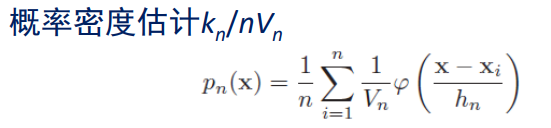我们发现，我们上面用的窗函数是一个超立方体内为1，我们希望推广，找到一些其他的窗函数，但要满足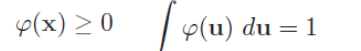即：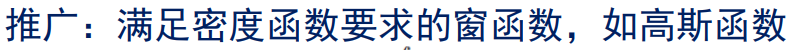为什么窗函数需要满足积分为1？其实主要是因为概率密度函数积分要为1，所以推得窗函数也要。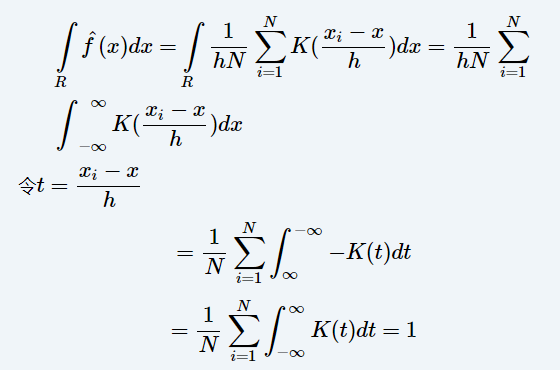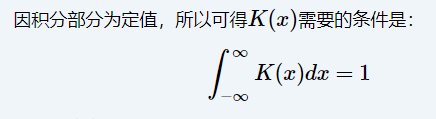除了之前的方窗函数，我们也经常选用如下形式的高斯窗函数。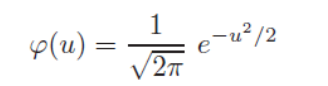然后用这个来估计样本概率密度。（我们先假设1维的情况下）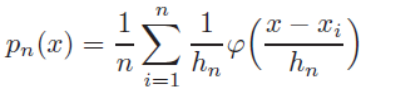例子：下面展示的是只有一个样本点，我们使用高斯窗函数，选用不同的窗口大小得到的估计结果。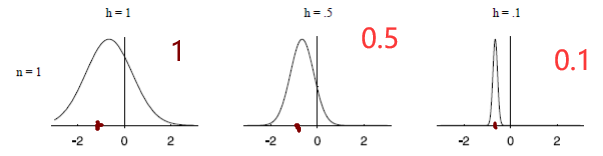请注意，这里的纵轴没有标刻度，实际上 h = 0.1 h=0.1 的时候函数是最高的，但是很窄，方差小。即：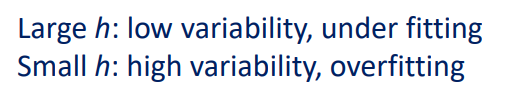例子：
假设有一批样本点，我们选定一个 h n h_n ，有如下结果。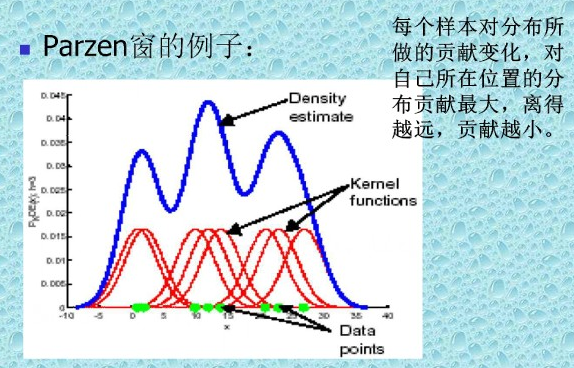解释：
显然，估计的方法是：对每一个样本点的头上套一个窗（红色），然后叠加起来求平均，就是最终的样本概率密度了（蓝色）。（上面展示的图是直接相加，没有求平均，为了好看而已）
显然上面的窗就是下面这个式子（对比 p n ( x ) p_n(x) 的表达式即可）：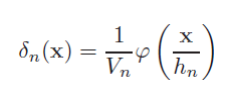注意到，这个式子积分也是为1的！自己可以推一下。
我们知道：直观上样本数量无穷大的时候，然后我们把窗口设得比较小的时候，可以拟合真实的概率密度函数，下面也有一个理论证明：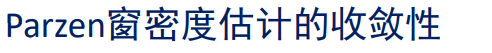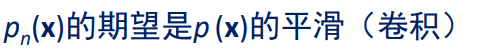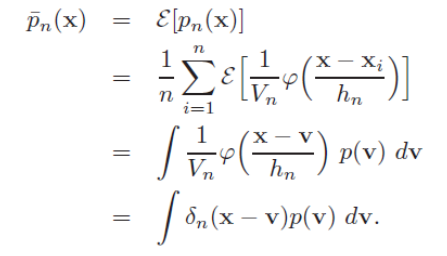上述求和除以n没有了是因为：得到上述的积分等式之后，我们有结论：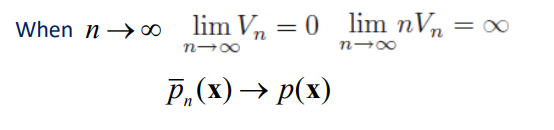我们继续举个分类的例子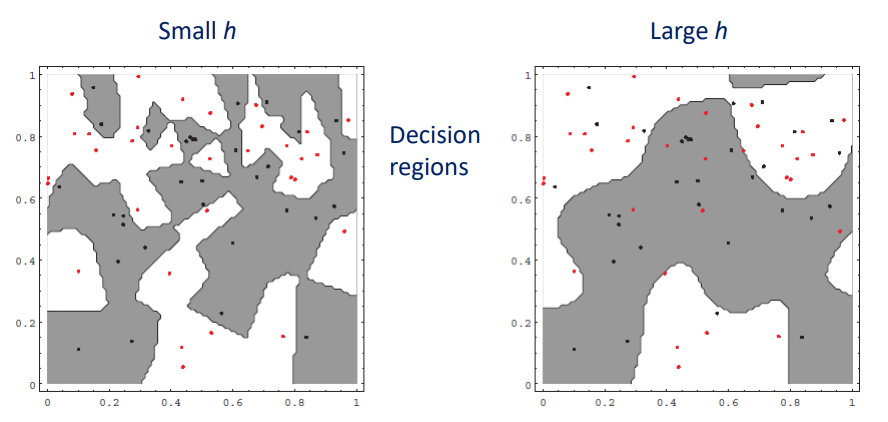使用parzen窗估计做法很简单，就是对每一类拟合一个样本概率密度函数，然后得到分界面如上所示。区别在于，在拟合样本概率密度的时候，选择窗口的大小不同会导致上面的不同，选择小的窗口可以带来过拟合。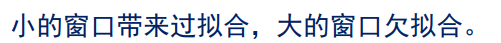改进办法：根据局部密度采用自适应窗口大小。K近邻估计

如何计算样本概率密度？个人觉得V也是一个与k有关的数，k越大，V也越大。
那么如何选择k呢？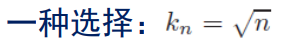例子：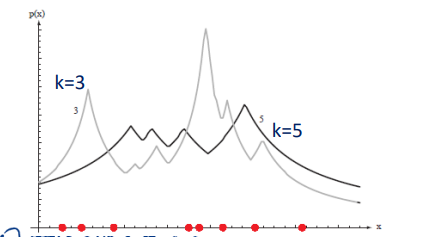有人难以理解，为什么是这样，我们可以假设k=1，那么变成最近邻，在任意样本点 x i x_i 上面，其概率密度函数为 p n ( x i ) = 1 / n 0 = ∞ p_n(x_i)=\frac{1/n}{0}=\infty 。即k越小越突兀。为什么 V n V_n 为0？因为对于 x i x_i 而言，最近的样本点是 x i x_i ，所以，在一维平面上，有 V n = x i − x i = 0 V_n=x_i-x_i=0 。即 V n V_n 是指最近的那个样本点到 x x 的距离的两倍（在一维中是距离或叫线段长度）。
一般地，一维中有：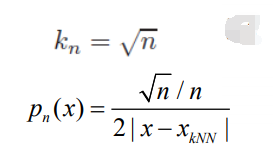x k N N x_{kNN} 是指离 x x 第k个最近的点。
拓展，在二维中 V n V_n 是圆的面积，三维中是球体的体积。
使用KNN来贝叶斯分类：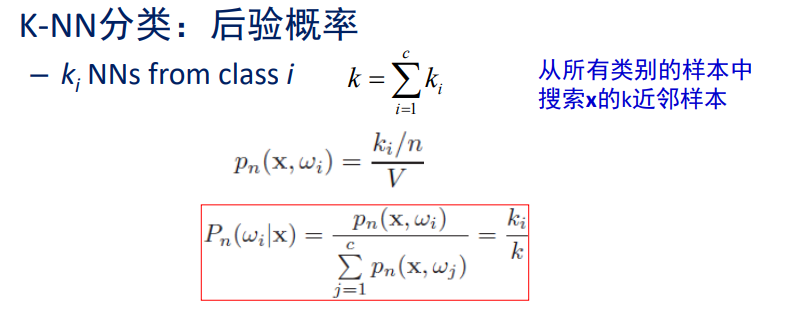非常精彩，红色公式中下面是 p ( x ) p(x) ，上面是 p ( x , w i ) p(x,w_i) 联合概率密度。而且，这和我们的投票方法是一样的， k i k_i 中谁大就分给谁。
当然还有使用KNN分类的办法，上面不是唯一的。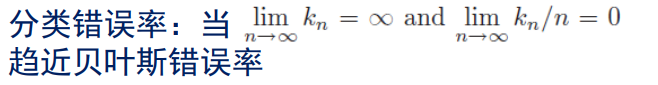原因很简单，因为 n n 趋于无穷的时候， p ( x ) p(x) 估计准确，所以趋近于贝叶斯错误率。
下面介绍一个特殊情况：最近邻分类。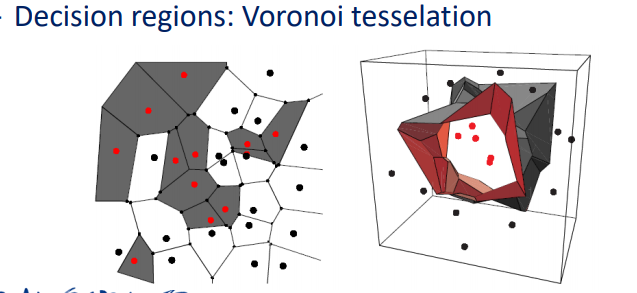K近邻的快速计算

先计算部分欧式距离，总共d维，先计算前r维的距离。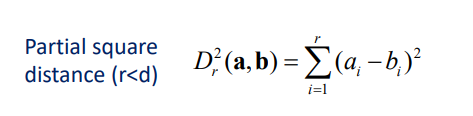假设:我们是快速计算最近邻。
那么快速计算的方法是：先计算x对第一个样本的全部距离，设为最小值，然后计算与第二个样本的部分距离，一旦超过最小值，后面的部分距离就不用算了。
再介绍一个思路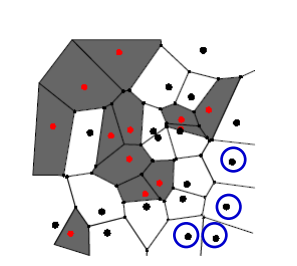即先将样本预处理，将圆形黑色删除，因为删除不会导致误判！

还有一些其他的算法，比如人工智能中很多非常重要的搜索算法，这里可能用得上！总之，应该意识到，快速计算非常重要，否则如果100万个点，k近邻很慢。

Parzen窗方法的快速计算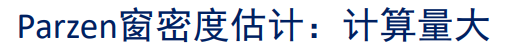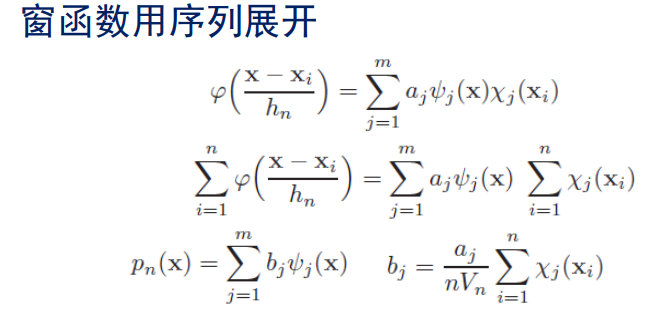其中：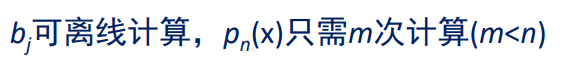这样，预测x的类别的时候就方便了。 b j b_j 早就算好了，算个 p n ( x ) p_n(x) 就行。
例子：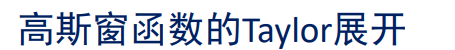展开到第2项。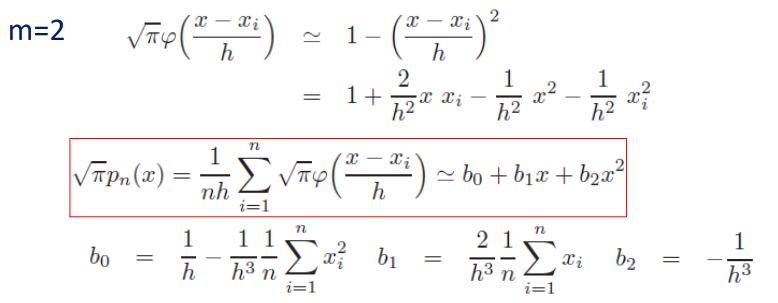从而提前计算好 b 1 , b 2 , b 0 b_1,b_2,b_0 即可。

总结

非参数估计和参数估计有联系但有很大区别，两者都非常重要。前者假定有一个概率密度函数形式，后者可以处理具有任意概率分布形式的数据。
讨论：非参数估计中，在我们对所有训练样本非参数估计后，按理可以得到一个概率密度函数，一般这个是一个具有非常多参数的函数，我们可以保存起来，然后丢弃所有样本，就像在参数估计一样。但是也要注意到，正文中两个快速计算方法都是基于局部空间临时计算概率密度，即所有样本都还要保留，可见这是另一种做法。

展开全文qq_43391414 2020-12-25 22:24:51
• 实验三 参数估计与非参数估计实验报告 python 机器学习

实验三：参数估计与非参数估计实验报告 1811377 李文浩 一、实验目的 通过本次实验实现机器学习中常用的参数估计和非参数估计的方法 使用编程加深对最大似然估计、最大后验概率估计等方法的认识 建立数据集学习...

实验三：参数估计与非参数估计实验报告

一、实验目的

1. 通过本次实验实现机器学习中常用的参数估计和非参数估计的方法
2. 使用编程加深对最大似然估计、最大后验概率估计等方法的认识
3. 建立数据集学习使用python对多元数据进行操作

二、代码框架

• 本次实验使用的函数框架如下:

1.Gaussian_function(x, mean, cov)
#计算多维(这里是2维)样本数据的概率p(x|w)，参数mean是已知的均值向量
#cov是已知的协方差矩阵，x是样本数据
2.Generate_Sample_Gaussian(mean, cov, P, label)
#生成符合正态分布的数据,P是指的先验概率,label是类标签
3.Generate_DataSet(mean, cov, P)
#根据先验概率生成数据集
4.Generate_DataSet_plot(mean, cov, P)
#画出不同先验对应的散点图
5.Likelihood_Test_Rule(X, mean, cov, P)
#似然率测试规则
6.Max_Posterior_Rule(X, mean, cov, P)
#最大后验概率规则
7.repeated_trials(mean, cov, P1, P2)
#单次实验求不同准则下的分类误差

三、代码详细说明

• Gaussian_function(x, mean, cov)
函数功能:计算p(x|w)

def Gaussian_function(x, mean, cov):
det_cov = np.linalg.det(cov)  # 计算方差矩阵的行列式
inv_cov = np.linalg.inv(cov)  # 计算方差矩阵的逆
#计算概率p(x|w)
p = 1/(2*np.pi*np.sqrt(det_cov))*np.exp(-0.5 *
np.dot(np.dot((x - mean),inv_cov), (x - mean)))
return p

这里使用了如下的公式: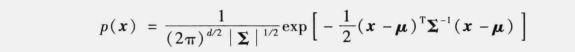d是指的数据的维数，这里是2;
Σ是指的协方差矩阵，这里是cov;
μ是指的均值向量这里是mean

np.linalg的三个函数:
1.np.linalg.det() 求矩阵的行列式
2.np.linalg.inv() 求矩阵的逆
3.np.dot() 求两矩阵的乘积

• Generate_Sample_Gaussian(mean, cov, P, label)
函数功能:生成符合正态分布的数据,P是指的先验概率,label是类标签

def Generate_Sample_Gaussian(mean, cov, P, label):
'''
mean 为均值向量
cov 为方差矩阵a
P 为单个类的先验概率
return 单个类的数据集
'''
# round(x[,n=0]) 保留到几位小数
temp_num = round(1000 * P)

# 生成一个多元正态分布矩阵
x, y = np.random.multivariate_normal(mean, cov, temp_num).T

#x,y坐标，x和y矩阵均符合正态分布
#z表示每个点属于哪一类
z = np.ones(temp_num) * label
X = np.array([x, y, z])

#X.T中每个元素都是有三个元素的列表，分别表示x值，y值，以及对应的标签
return X.T

multivariate_normal(mean, cov, size=None, check_valid=None, tol=None)

1.函数功能:生成一个多元正态分布矩阵
2.mean:正态分布的均值向量
3.cov:正态分布的协方差矩阵
4.size:矩阵的大小(数量，维度)
5.check_valid:如果cov不是半正定矩阵的处理方法,一共有三个值:warn(输出警告,仍出结果),raise(报错),ignore(或略错误)
6.tol:检查协方差矩阵奇异值时的公差

• Generate_DataSet(mean, cov, P)
函数功能:根据先验概率生成数据集

def Generate_DataSet(mean, cov, P):
# 按照先验概率生成正态分布数据
# 返回所有类的数据集
X = []
label = 1
for i in range(3):
# 把此时类i对应的数据集加到已有的数据集中
X.extend(Generate_Sample_Gaussian(mean[i], cov, P[i], label))
label += 1
i = i + 1
return X

注意这里extend()append()的区别:
1.append将括号中的参数作为一个元素添加到列表对象的后面，即便参数是一个列表也是同样
2.extend将不是序列类型(list，dict，tuple)的参数作为一个元素添加到列表对象的后面，如果是序列类型的参数，就将序列和列表合并放在列表的后面

• Generate_DataSet_plot(mean, cov, P)
函数功能:画出不同先验对应的散点图

def Generate_DataSet_plot(mean, cov, P):
# 画出不同先验对应的散点图
xx = []
label = 1
# 将xx变为包含三类数据的数据集
for i in range(3):
xx.append(Generate_Sample_Gaussian(mean[i], cov, P[i], label))
label += 1
i = i + 1
#在这时xx是一个有三个元素的列表，每个元素都是一个类
# 画图
plt.figure()
if P==[1 / 3, 1 / 3, 1 / 3]:
plt.title("X1分布图")
else:
plt.title("X2分布图")
for i in range(3):
#画出每类的样本向量(x,y)
plt.plot(xx[i][:, 0], xx[i][:, 1], '.', markersize=4.)
#画出每类的中心点(均值向量对应的点)
plt.plot(mean[i], mean[i], 'r*')
plt.show()#没有这句图像不显示
return xx

• Likelihood_Test_Rule(X, mean, cov, P)
函数作用:使用似然率测试规则进行估计得准确率

# 似然率测试规则
def Likelihood_Test_Rule(X, mean, cov, P):
class_num = mean.shape  # 类的个数
num = np.array(X).shape
error_rate = 0
for i in range(num):
p_temp = np.zeros(3)
for j in range(class_num):
# 计算样本i决策到j类的概率
p_temp[j] = Gaussian_function(X[i][0:2], mean[j], cov)
p_class = np.argmax(p_temp) + 1  # 得到样本i决策到的类
if p_class != X[i]:
error_rate += 1
return round(error_rate / num , 3)

这个函数分别计算数据集中的每个样本被决策到三个不同的类的概率p_temp,使用np.argmax()求出列表中值最大的元素的下标index，index+1即为我们决策出的结果p_class，用p_class和样本真正的类别X[i]比较，判断正确与否，计算准确率

• Max_Posterior_Rule(X, mean, cov, P)
函数功能:使用最大后验概率规则进行估计得准确率

##最大后验概率规则
def Max_Posterior_Rule(X, mean, cov, P):
class_num = mean.shape  # 类的个数
num = np.array(X).shape
error_rate = 0
for i in range(num):
p_temp = np.zeros(3)
for j in range(class_num):
# 计算样本i是j类的后验概率
p_temp[j] =Gaussian_function(X[i][0:2],mean[j],cov)*P[j]
p_class = np.argmax(p_temp) + 1  # 得到样本i分到的类
if p_class != X[i]:
error_rate += 1
return round(error_rate / num,3)

基本思路和似然率测试规则是一样的，但这里计算概率的函数是P(x|w)*P[i]

• repeated_trials(mean, cov, P1, P2)
函数功能:单次实验求不同准则下的分类误差

# 单次试验求不同准则下的分类误差
def repeated_trials(mean, cov, P1, P2):
# 根据mean，cov，P1,P2生成数据集X1,X2
# 通过不同规则得到不同分类错误率并返回
# 生成N=1000的数据集
X1 = Generate_DataSet(mean, cov, P1)
X2 = Generate_DataSet(mean, cov, P2)
error = np.zeros((2, 2))
# 计算似然率测试规则误差
error_likelihood = Likelihood_Test_Rule(X1, mean, cov, P1)
error_likelihood_2 = Likelihood_Test_Rule(X2, mean, cov, P2)
error = [error_likelihood, error_likelihood_2]
# 计算最大后验概率规则误差
error_Max_Posterior_Rule = Max_Posterior_Rule(X1, mean, cov, P1)
error_Max_Posterior_Rule_2 = Max_Posterior_Rule(X2, mean, cov, P2)
error = [error_Max_Posterior_Rule, error_Max_Posterior_Rule_2]
return error

分别生成两组数据集，分别对这两个数据集进行不同规则下的分类误差分析，将结果存在error中并返回

1.基本要求

在两个数据集合上分别应⽤“似然率测试规则” 、“最⼤后验概率规则” 进⾏分类实验，计算分类错误率，分析实验结果。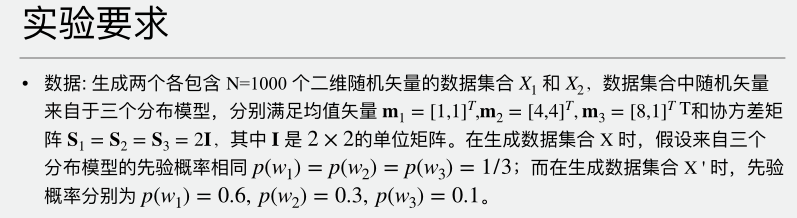• 1.根据题设设置我们所需要用到的参数

mean = np.array([[1, 1], [4, 4], [8, 1]])  # 均值数组
cov = [[2, 0], [0, 2]]  # 协方差矩阵
num = 1000  # 样本个数
P1 = [1 / 3, 1 / 3, 1 / 3]  # 样本X1的先验概率
P2 = [0.6, 0.3, 0.1]  # 样本X2的先验概率

• 2.绘制散点图，观察样本分布

Generate_DataSet_plot(mean, cov, P1)  # 画X1数据集散点图
Generate_DataSet_plot(mean, cov, P2)  # 画X2数据散点图

结果如下: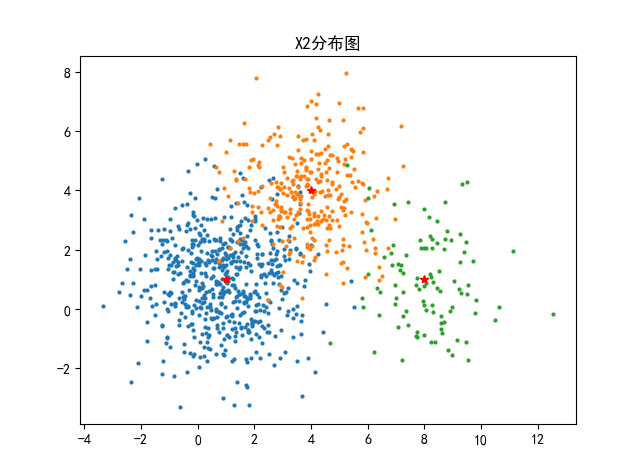• 3.进行十次试验观察试验结果

# 计算十次运算的总误差
error_all = np.zeros((2, 2))
# 测试times_num次求平均
times_num = 10
for times in range(times_num):
error=repeated_trials(mean,cov,P1,P2)
print("第{}次试验: 极似然规则 最大后验规则".format(times+1))
print("X1误差:   \t{}  \t{}".format(error,error))
print("X2误差:   \t{}  \t{}".format(error, error))
error_all += error
# 计算平均误差
error_ave = np.around(error_all / times_num,4)
print("平均误差:  极似然规则   最大后验规则")
print("X1误差:  \t{}   \t{}".format(error_ave,error_ave))
print("X2误差:  \t{}   \t{}".format(error_ave,error_ave))

结果如下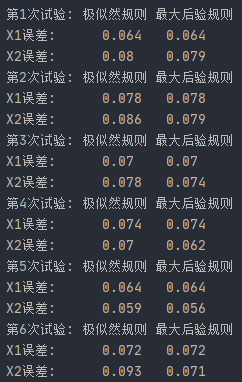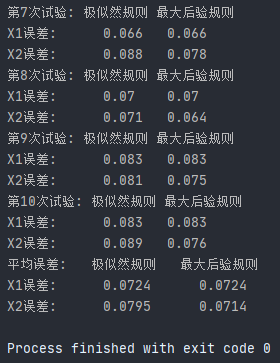分析试验结果：

1.当每个类的先验概率P相同或差别不大时，及似然率测试规则和最大后验概率规则分类结果相差不大
2.当先验概率相差较大时，及似然率规则更好一些

展开全文joachimq 2021-04-12 15:28:43
• 参数估计之矩估计 统计学

介绍参数估计中点估计的常用方法：矩估计法。并通过例题加深理解

1. 点估计定义

• 点估计定义

x 1 , x 2 , ⋯   , x n x_1,x_2,\cdots,x_n 是来自总体的一个样本，用于估计位置参数 θ \theta 的统计量 θ ^ = θ ^ ( x 1 , x 2 , ⋯   , x n ) \hat{\theta}=\hat{\theta}(x_1,x_2,\cdots,x_n) 称为 θ \theta 的估计量，或称为 θ \theta 点估计，简称估计

• 构造估计量的方式有很多，常用的有矩估计法最大似然估计法

2. 矩估计法

• 矩估计法简单点的说，就是用样本的矩，替换(估计)总体的矩。

记总体 k k 阶原点矩为 μ k = E ( X k ) \mu_k=E(X^k)

样本 k k 阶原点矩为 A k = 1 n ∑ i = 1 n ( X i k ) A_k=\frac{1}{n}\sum\limits_{i=1}^n (X_i^k)

记总体 k k 阶中心矩为 v k = E [ ( X − E ( X ) ) k ] v_k=E[(X-E(X))^k]

样本 k k 阶中心矩为 B k = 1 n ∑ i = 1 n ( X i − X ‾ ) k B_k=\frac{1}{n}\sum\limits_{i=1}^n (X_i-\overline{X})^k

3. 通过例题理解矩估计法

• 例1，设总体 X X 的均值 μ \mu 和方差 σ 2 \sigma^2 都存在，且有 σ 2 > 0 \sigma^2>0 ，但 μ , σ 2 \mu,\sigma^2 均为未知，又设 X 1 , X 2 , ⋯   , X n X_1,X_2,\cdots,X_n 为来自 X X 的样本，试求 μ , σ 2 \mu,\sigma^2 的矩估计量。

解：

样本的一阶矩 为 X ‾ \overline{X} ，总体的一阶矩为 E ( X ) = μ E(X)=\mu

因此有 μ ^ = X ‾ \hat{\mu} = \overline{X}

样本的二阶矩为 A 2 = 1 n ∑ i = 1 n X i 2 A_2=\frac{1}{n}\sum\limits_{i=1}^n X_i^2 ，总体的一阶矩为 E ( X 2 ) E(X^2)

则有 E ( X 2 ) = 1 n ∑ i = 1 n X i 2 E(X^2)=\frac{1}{n}\sum\limits_{i=1}^n X_i^2

由于 D ( X ) = E ( X 2 ) − ( E X ) 2 D(X)=E(X^2)-(EX)^2

因此有

σ 2 ^ = 1 n ∑ i = 1 n X i 2 − X ‾ 2 = 1 n ∑ i = 1 n ( X i − X ‾ ) 2 \begin{aligned}\hat{\sigma^2} &= \frac{1}{n}\sum\limits_{i=1}^n X_i^2 - \overline{X}^2 \\&= \frac{1}{n}\sum\limits_{i=1}^n(X_i-\overline{X})^2 \end{aligned}

• 例2，设离散型随机变量 X X ，其分布律如下

X 1 2 3 P θ 2 2 θ ( 1 − θ ) ( 1 − θ ) 2 \begin{array}{c|lc} X & 1 & 2 &3 \\ \hline P & \theta^2 & 2\theta(1-\theta) & (1-\theta)^2 \end{array}

X X 中取得样本 1 , 2 , 1 {1,2,1} ，求 θ \theta 的矩估计量

解：样本一阶矩 A 1 = 1 3 ( 1 + 2 + 1 ) = 3 4 A_1 = \frac{1}{3}(1+2+1) = \frac{3}{4}

总体一阶矩 μ 1 = 1 ∗ θ 2 + 2 ∗ 2 θ ( 1 − θ ) + 3 ( 1 − θ ) 3 \mu_1 = 1*\theta^2+2*2\theta(1-\theta)+3(1-\theta)^3

μ 1 = A 1 \mu_1 =A_1 ，很容易解出 θ ^ = 5 6 \hat {\theta}= \frac{5}{6}

4. 矩估计优缺点

• 优点
1. 矩估计法原理简单、使用方便，使用时可以不知总体的分布，而且具有一定的优良性质
2. 样本数量足够大时，矩估计的优势也就越明显
• 缺点
1. 当总体类型已知时，没有充分利用分布提供的信息，因此矩估计不一定是理想估计
2. 样本数较少时，矩估计的结果将非常糟糕
3. 一般场合下，矩估计不具有唯一性(关于这一点，后面我们会介绍估计值的优良性准则)
4. 矩估计应用的前提是总体的矩存在
展开全文SpiritedAway1106 2021-01-15 09:12:07
• weixin_42551967 2021-02-06 13:19:38
• 非参数估计—Parzen窗与K-nearest-neighbor 算法 机器学习

qq_45785407 2021-11-16 15:43:51
• walking_visitor 2021-04-21 10:16:10
• as604049322 2021-04-03 01:15:43
• qq_40947195 2021-08-12 20:13:41
• weixin_39559097 2020-12-22 16:57:16
• 代码：非参数密度估计的三种python实现 (直方图，核密度估计，K近邻) python 数据挖掘 无监督学习

weixin_42388833 2021-06-06 17:31:40
• weixin_33608494 2021-01-12 10:42:28
• 收藏 | 神经网络的计算量和参数量估计总结 卷积 算法 深度学习 人工智能 神经网络

qq_15698613 2021-05-13 16:27:36
• 使用python的模拟退火算法估计heston期权定价模型的五个参数（新） python 模拟退火算法 开发语言

weixin_45590329 2021-08-11 16:22:23
• weixin_39887386 2020-12-19 14:23:04
• rust的虚类型参数 rust 开发语言 后端

fpcc 2021-12-06 15:07:01
• weixin_39824020 2021-01-17 18:45:49
• u012958850 2021-05-16 10:34:55
• weixin_39529914 2021-03-17 15:46:17
• 贝叶斯网专题11：参数学习之极大似然估计 机器学习 人工智能

deepbodhi 2021-10-05 19:10:40
• 基础：神经网络的计算量和参数量估计总结 卷积 算法 神经网络 计算机视觉 机器学习

lgzlgz3102 2021-01-25 11:00:46
• 机器学习（十八）极大似然估计 自然语言处理 自动驾驶 数据挖掘 机器学习

yanqianglifei 2021-02-27 23:27:18
• qq_42374697 2020-12-28 20:20:15
• weixin_36057373 2021-04-19 01:40:26
• 贝叶斯优化-最大似然估计函数参数化(2/3) 机器学习 朴素贝叶斯算法

weixin_43423614 2021-06-27 14:48:49
• weixin_28692817 2021-01-19 04:36:11
• weixin_33288893 2021-02-03 00:43:09
• MaoziYa 2021-03-31 08:54:46
• weixin_29696999 2020-12-31 09:38:58
• c85759351 2021-01-19 14:14:52...